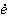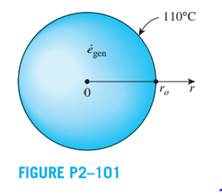#### Service

Chat Now

Consider a homogeneous spherical piece of radioactive material of radius ro = 0.04 m that is generating heat at a constant rate ofgen = 5 × 107 W/m3. The heat generated is dissipated to the environment steadily. The outer surface of the sphere is maintained at a uniform temperature of 110°C and the thermal conductivity of the sphere is k = 15 W/m·K. Assuming steady one-dimensional heat transfer,

(a) express the differential equation and the boundary conditions for heat conduction through the sphere,

(b) obtain a relation for the variation of temperature in the sphere by solving the differential equation, and

(c) determine the temperature at the center of the sphere.rahul 21-Jul-2020Get solution